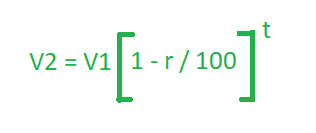Related Articles

# Program to find the Depreciation of Value

• Last Updated : 27 Apr, 2021

The value of any article or item subject to wear and tear, decreases with time. This decrease is called its Depreciation. Given three variable V1, R and T where V1 is the initial value, R is the rate of depreciation and T is the time in years. The task is to find the value of the item after T years.
Examples:

Input: V1 = 200, R = 10, T = 2
Output: 162
Input: V1 = 560, R = 5, T = 3
Output: 480.13

Approach: As in Compound Interest, interest is regularly added to the principal at the end of the agreed intervals of time to generate a new and fresh principal. Similarly, Depreciated value is the decreased value from the amount at the end of agreed intervals of time to generate a new Value.
Thus if V1 is the value at a certain time and R% per annum is the rate (the rate can not be more than 100%) of depreciation per year, then the value V2 at the end of T years is:Below is the implementation of the above approach :

## C++

 `// CPP program to find depreciation of the value``// initial value, rate and time are given``#include ``using` `namespace` `std;` `// Function to return the depreciation of value``float` `Depreciation(``float` `v, ``float` `r, ``float` `t)``{` `    ``float` `D = v * ``pow``((1 - r / 100), t);` `    ``return` `D;``}` `// Driver Code``int` `main()``{``    ``float` `V1 = 200, R = 10, T = 2;` `    ``cout << Depreciation(V1, R, T);` `    ``return` `0;``}`

## Java

 `// Java program to find depreciation of the value``// initial value, rate and time are given``import` `java.io.*;` `class` `GFG``{` `// Function to return the depreciation of value``static` `float` `Depreciation(``float` `v,``                          ``float` `r, ``float` `t)``{``    ``float` `D = (``float``)(v * Math.pow((``1` `- r / ``100``), t));` `    ``return` `D;``}` `// Driver code``public` `static` `void` `main(String[] args)``{``    ``float` `V1 = ``200``, R = ``10``, T = ``2``;``    ` `    ``System.out.print(Depreciation(V1, R, T));``}``}` `// This code is contributed by anuj_67..`

## Python3

 `# Python 3 program to find depreciation of the value``# initial value, rate and time are given``from` `math ``import` `pow` `# Function to return the depreciation of value``def` `Depreciation(v, r, t):``    ``D ``=` `v ``*` `pow``((``1` `-` `r ``/` `100``), t)` `    ``return` `D` `# Driver Code``if` `__name__ ``=``=` `'__main__'``:``    ``V1 ``=` `200``    ``R ``=` `10``    ``T ``=` `2` `    ``print``(``int``(Depreciation(V1, R, T)))` `# This code is contributed by``# Surendra_Gangwar`

## C#

 `// C# program to find depreciation of the value``// initial value, rate and time are given``using` `System;` `class` `GFG``{` `// Function to return the depreciation of value``static` `float` `Depreciation(``float` `v, ``float` `r, ``float` `t)``{` `    ``float` `D = (``float``) (v * Math.Pow((1 - r / 100), t));` `    ``return` `D;``}` `// Driver code``public` `static` `void` `Main()``{``    ``float` `V1 = 200, R = 10, T = 2;``    ` `    ``Console.WriteLine(Depreciation(V1, R, T));``}``}` `// This code is contributed by nidhiva`

## Javascript

 `// javascript program to find depreciation of the value``// initial value, rate and time are given` `// Function to return the depreciation of value`` ``function` `Depreciation( v,  r,  t)``{``  ` `    ``var` `D =  v * Math.pow((1 - r / 100), t)``    ``return` `D;``}``  ` `// Driver code``    ``var` `V1 = 200,  R = 10,  T = 2;``    ``document.write(Depreciation(V1, R, T));` `// This code is contributed by bunnyram19. `
Output:
`162`

Attention reader! Don’t stop learning now. Get hold of all the important DSA concepts with the DSA Self Paced Course at a student-friendly price and become industry ready.  To complete your preparation from learning a language to DS Algo and many more,  please refer Complete Interview Preparation Course.

In case you wish to attend live classes with experts, please refer DSA Live Classes for Working Professionals and Competitive Programming Live for Students.

My Personal Notes arrow_drop_up